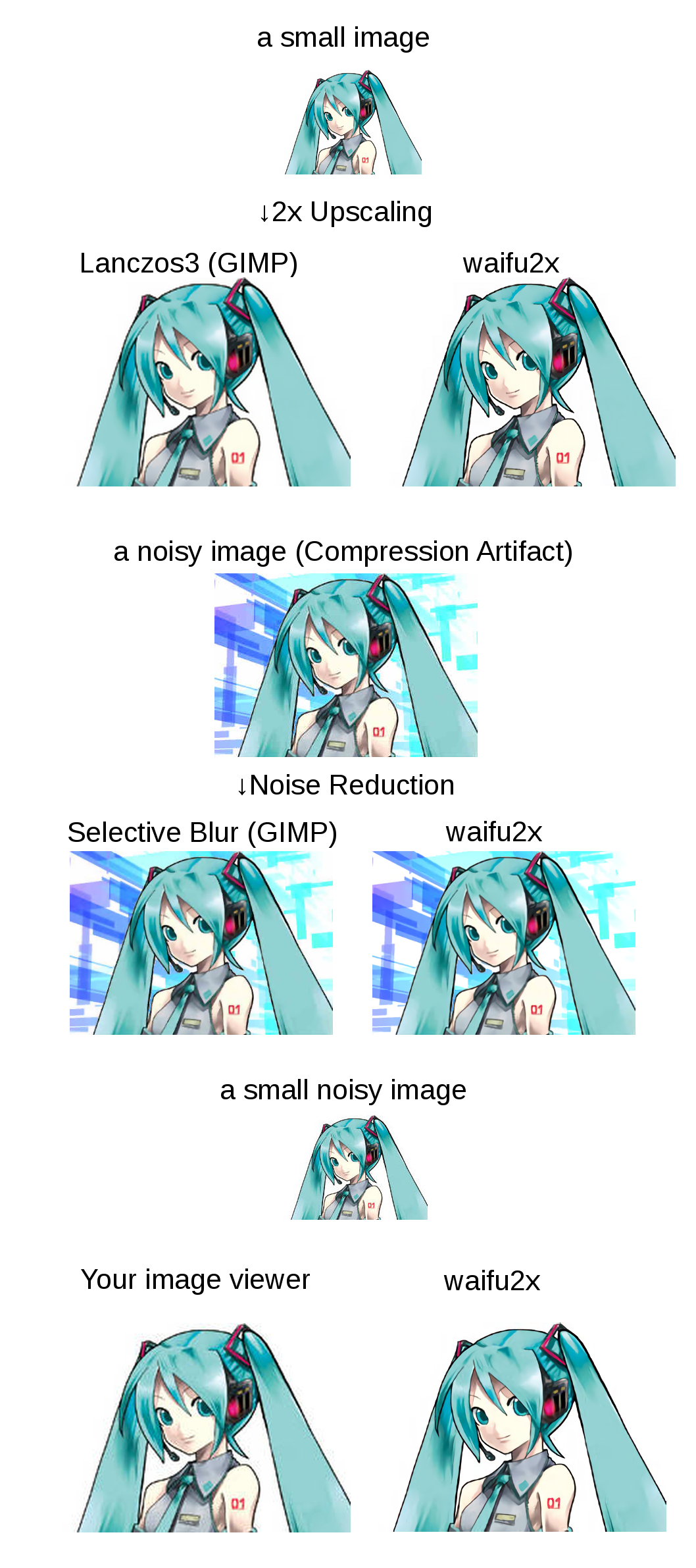# Source code reading of waifu2x

Memo for self study.

## SRCNN – Super resolution by deep convolutional neural network

Recently many application is developed using deep learning. waifu2x is a image super resolution application using convolutional neural network.Cite from waifu2x

The source code is open at github. It is developed using torch7, so the Lua programming language is used. I have never used Lua, but it is similar to python, so reading Lua source code was not so difficult without further study.

waifu2x supports upscaling (“scale”) and noise reduction (“noise”), but I will focus on scaling function here.
* Actually same CNN network architecture is used for upscaling, and noise reduction, they work in same way. Main difference is only training set used during training.

Theory of super resolution using convolutional neural network (SRCNN) is first introduced in below paper

## Model

The Convolutional neural network (CNN) model is defined at `waifu2x/lib/srcnn.lua`

waifu2x/lib/srcnn.lua

where `ch` is a input/output channel number, `ch = 1` when you train only Y channel of YCbCr image, and `ch = 3` can be used when RGB image is trained.

So basically it is composed of 7 depth of convolutioal layer with LeakyReLU activation. LeakyReLU is also called PReLU, Parametric Rectified Linear Unit.

## Image converting process

Next, let’s see how to preprocess, model forward propagate, postprocessing image is going on. The main process of image converting is written in waifu2x.lua,  but its main point is

so main processing is done at `reconstruct.scale` function at `/lib/reconstruct.lua`

`x`,` `image array is just passed to` scale_y `function

We will separate 3 parts here, splitted at the line 22, `reconstruct_y(model, x, offset, block_size)`

• Preprocessing: preparation of input `x `for the model,
• Model forwarding: input `x` to the CNN model and get output `y`, it is done at `reconstruct_y(model, x, offset, block_size)`
• Postprocessing: some postprocess to convert obtained output `y` to image.

### Preprocess

We will input only Y channel to the model, and the size of the image is already scaled before inputting to the model,

Important part is

1. Upscaling x using nearest neighbor method,

2. Convert image from RGB to YUV, and input only Y channel (`x`) to the model

### Model forwarding

It is done in `reconstruct_y` function

input `x` and getting output `new_x` by using `model:forward`

### Postprocess

1. Normalization: clipping calculated output y between 0 – 1.

the input image `x` was normalized, and its range is 0 – 1, but model may output the value outside this range. It is clipped by

2. Merging `x_lanchos` (UV channel) and `y` (Y channel)

waifu2x only upscales Y channel using SRCNN, and UV channel upscaling is done by conventional Lanchos method.

3. Revert from YUV to RGB

## Training

• ADAM is used for MSGD parameter tuning.
• Huber loss is used for loss function

### loss function

• clipped: clipping input 0 – 1, to compare with the target data.
• weighted: weight used for calculating loss for each channel (RGB), but it is not important for only Y channel training.
• Huber loss: compared to MSE (minimum squared error), it is less sensitive to outliers.
ref: wikipedia